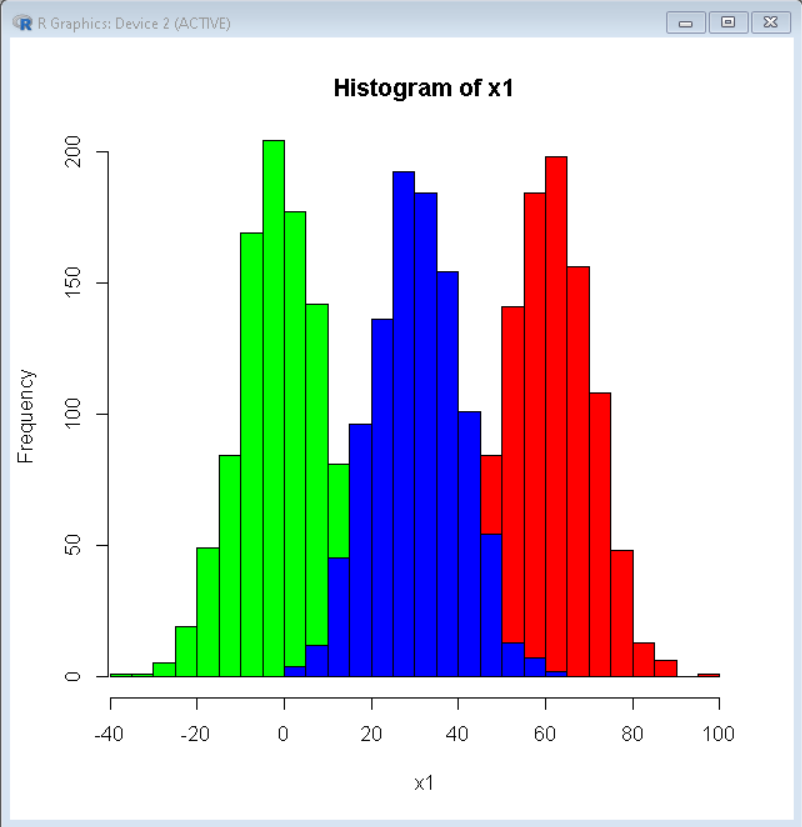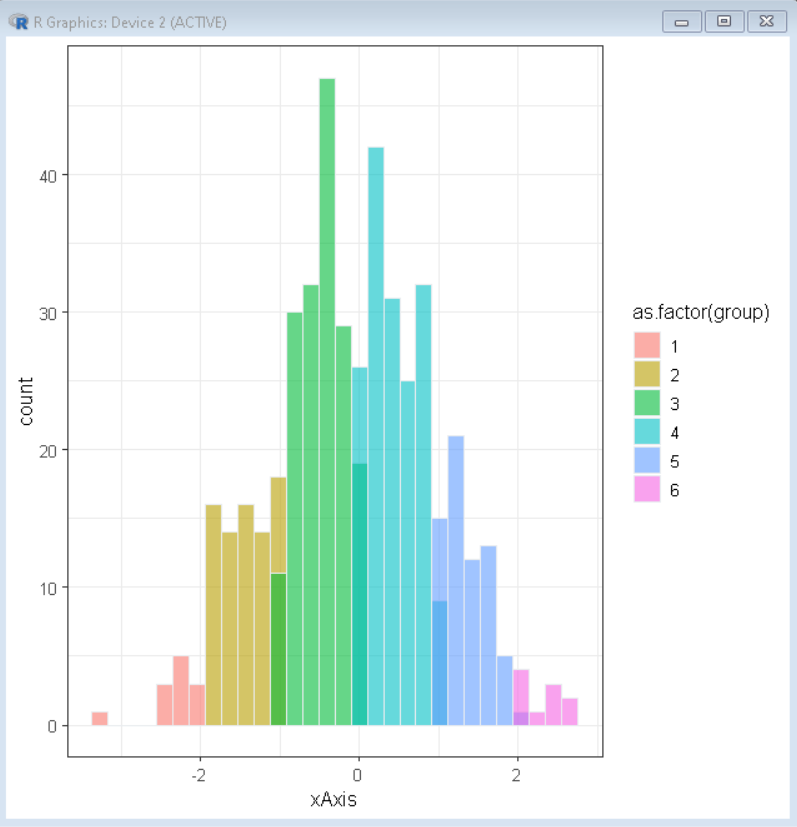# How to Plot Multiple Histograms in R?

• Last Updated : 19 Dec, 2021

In this article, we will discuss how to plot multiple histograms in the R Programming language.

## Method 1: Multiple Histogram in Base R

To create multiple histograms in base R, we first make a single histogram then add another layer of the histogram on top of it. But in doing so some plots may clip off as axis are made according to the first plot. So, we can add the xlim, and the ylim parameters in the first plot to change the axis limit according to our dataset.

Syntax:

```hist( data, col, xlim, ylim )
hist( data, col )```

where,

• data: determines the data vector to be plotted.
• xlim: determines the vector with x-axis limit.
• ylim: determines the vector with y-axis limit.
• col: determines the color of bars of the histogram.

Example:

Here, is basic multiple histograms made in the base R Language with help of hist() function.

## R

 `# create data vector ` `x1 = ``rnorm``(1000, mean=60, sd=10) ` `x2 = ``rnorm``(1000, mean=0, sd=10) ` `x3 = ``rnorm``(1000, mean=30, sd=10) ` ` `  `# create multiple histogram ` `hist``(x1, col=``'red'``, xlim=``c``(-35, 100)) ` `hist``(x2, col=``'green'``, add=``TRUE``) ` `hist``(x3, col=``'blue'``, add=``TRUE``)`

Output:## Method 2: Multiple Histogram Using ggplot2

To create multiple histograms in ggplot2, we use ggplot() function and geom_histogram() function of the ggplot2 package. To visualize multiple groups separately we use the fill property of aesthetics function to color the plot by a categorical variable.

Syntax:

`ggplot( df, aes( x, fill ) ) + geom_histogram( color, alpha ) `

where,

• df: determines the data frame to be plotted.
• x: determines the data variable.
• fill: determines the color of bars in the histogram.
• color: determines the color of the boundary of bars in the histogram.
• alpha: determines the transparency of the plot.

Example:

Here, is basic multiple histograms made by using the geom_histogram() function of the ggplot2 package in the R Language.

## R

 `# load library ggplot2 ` `library``(ggplot2) ` `  `  `# set theme ` `theme_set``(``theme_bw``(12)) ` ` `  `# create x vector ` `xAxis <- ``rnorm``(500)              ` ` `  `# create groups in variable using conditional  ` `# statements ` `group <- ``rep``(1, 500)               ` `group[xAxis > -2] <- 2 ` `group[xAxis > -1] <- 3 ` `group[xAxis > 0] <- 4 ` `group[xAxis > 1] <- 5 ` `group[xAxis > 2] <- 6 ` ` `  `# create sample data frame ` `sample_data <- ``data.frame``(xAxis, group)  ` `  `  `# create histogram using ggplot()  ` `# function colored by group ` `ggplot``(sample_data, ``aes``(x=xAxis, fill = ``as.factor``(group)))+ ` `   ``geom_histogram``( color=``'#e9ecef'``, alpha=0.6, position=``'identity'``)`

Output:My Personal Notes arrow_drop_up
Recommended Articles
Page :